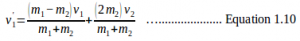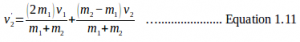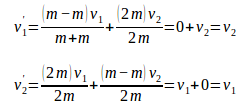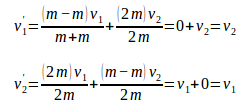# Perfectly elastic collisions

A collision of two objects is called a perfectly elastic collision if the momentum or kinetic energy of each object before the collision is equal to the momentum and kinetic energy of each object after the collision. In other words, the conservation of momentum law and conservation of kinetic energy law are applicable in perfectly elastic collisions. The use of the word elastic signifies that after the collision, the two objects do not stick together or are not attached to each other but bounce off. The momentum of each object is conserved.

The momentum of each object is conserved.

m1 v1 + m2 v2 = m1 v1 ’ + m2 v2 ’ ………………….. Equation 1.5

The kinetic energy of each object is conserved.

1⁄2 m v12 + 1⁄2 m v22 = 1⁄2 m v12 + 1⁄2 m v22 ………………….. Equation 1.6

[irp]

The perfectly elastic collision must be silent and does not generate heat due to friction between the two colliding objects. If the collision of two objects generates noise and heat, the kinetic energy of the objects is not conserved. Some kinetic energy is converted into sound energy and heat energy, and some are converted into internal energy. An example of a perfectly elastic collision is the collision of atomic and subatomic particles.

In a problem of perfectly elastic collision, if the initial speed is known while the final speed is unknown, the problem cannot be solved by only using equations 1.5 and 1.6. For this reason, both of the equations are manipulated to derive other equations, which can be used to determine the final speed.

Remove factor 1/2 then manipulate 1.6

m1 v12 + m2 v22 = m1 v1 2 + m2 v2 2

m1 v12 – m1 v12 = m2 v22 – m2 v22

m1 (v12 – v12 ) = m2 (v22 – v2 2) —> (a + b)(a – b) = a2 – b2

m1 (v1 + v1 ’) (v1 – v1 ’) = m2 (v2 ’ + v2 ) (v2 ’ – v2 ) ………………….. Equation 1.7

[irp]

Manipulate equation 1.5

m1 v1 – m1 v1 ’ = m2 v2 ’- m2 v2

m1 (v1 – v1 ’) = m2 (v2 ’ – v2) ………………….. Equation 1.8

Devide equation 1.7 by equation 1.8 to obtain the final result

(v1 + v1 ’) = (v2 ’ + v2)

v1 – v2 = v2 ’ – v1

v1 – v2 = – (v1 ’ – v2 ’) ………………….. Equation 1.9

Equations 1.5 and 1.9 can be used to solve problems of perfectly elastic collisions. Combine equations 1.5 and 1.9 to obtain two equations for determining the final velocities of two objects if their masses and initial velocities are unknown.When solving questions using the equations above, use the correct sign for v1 and v2. If object 1 moves to the right, v1 is positive, and conversely, if object two moves to the left, v2 is negative. If both objects move in different directions, but there is no information regarding the motion directions, v1 and v2 must be signed differently, for example, v1 is positive, and v2 is negative.

4.1.1 Two objects of the same massIf m1 = m2, v1 ’ = v2 and v2’ = v1 .

What if the two objects move in different directions?

For instance, if before collision object 1 moves to the right and object 2 moves to the left, object 1 will move to the left (v1’ = – v2) and object 2 will move to the right (v2’ = v1) after the collision.

What if either of the objects is initially at rest?

For instance, if before collision object 2 is at rest (v2 = 0), object 1 will be at rest (v1’ = 0) and object 2 will move at the same velocity as the initial velocity of object 1 (v2’ = v1) after collision. If before collision object 1 moves to the right, object 2 will move to the right after the collision. Hence, the two objects exchange velocities.

[irp]

Example question 4

Objects A (2 kg) and B (2 kg) move to opposite directions at speeds of 4 m/s and 2 m/s, respectively. If objects A and B collide in a perfectly elastic collision, what are the final speeds of objects A and B?

Known :

mA = 2 kg, mB = 2 kg, vA = 4 m/s, vB = – 2 m/s

Wanted: vA’ and vB

Solution :

vA’ = – 2 m/s and vB’ = 4 m/s

After the collision, object A moves at a speed of 2 m/s and object B moves at a speed of 4 m/s in opposite directions. If before collision object A moves to the right and object B moves to the left, object A will move to the left and object B will move to the right after the collision.

Example question 5

Object A (2 kg) moves to the right at a speed of 2 m/s and collides with object B (2 kg) which is at rest. If the two objects collide in a perfectly elastic collision, what are the final speeds of objects A and B?

Known :

mA = 2 kg, mB = 2 kg, vA = 2 m/s, vB = 0 m/s

Wanted: vA ’ and vB

Solution :

vA’ = 0 and vB’ = 2 m/s

After the collision, object A is at rest, and object B moves to the right at a speed of 2 m/s.

[irp]

4.1.2 Two objects of different masses

If two objects have different initial velocities and masses (minor difference), the final velocities are known by using equations 1.10 and 1.11.

If initially object 2 is at rest (v2 = 0), equation 1.10 becomes equation 1.12 and equation 1.11 becomes equation 1.13.If v2 = 0, m1 is very great, and m2 is very small, by solving equations 1.12 and 1.13 v1’ = v1 and v2’ = 2v1 are obtained. If v2 = 0, m1 is very small, and m2 is very great, by solving equations 1.12 and 1.13 v1 ’ = -v1 and v2 ’ = 0 are obtained. Positive and negative marks indicate opposite motion directions.

Example question 6

An object with a mass of 1 kg moves at a speed of 20 m/s and collides with a wall in a perfectly elastic collision. What are the final speeds of the object and the wall?

Known :

mA = 1 kg, vA = 20 m/s, mB = very great, vB = 0

Wanted : vA’ and vB

Solution :

vA’ = -20 m/s and vB’ = 0

After collision, object A bounces off at a speed of 20 m/s and object B remains at rest. If before collision object A moves to the right, it moves to the left after collision.